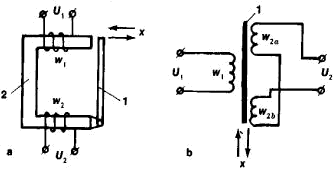# Transformer Transducer

The following article is from The Great Soviet Encyclopedia (1979). It might be outdated or ideologically biased.

## Transformer Transducer

a measuring transducer for the measurement of mechanical quantities, such as displacement, force, and angle of rotation, according to the change in the transformation ratio of a transformer or in the mutual induction between the transformer’s primary and secondary windings. [The Russian term transformatornyi datchik is used collectively to refer to two types of devices, which are known in English as the variable-reluctance transducer and the differential transformer.]

The operation of a variable-reluctance transducer (also called a linear variable-reluctance transducer, or LVRT) is based on the dependence of the electromotive force induced in the secondary winding on one of the factors mentioned above. These factors change as a result of a change in the gap in the core of the transformer, a change in the relative position of the windings, and so on. A diagram of a very simple variable-reluctance transducer, in which the gap in the core varies with the displacement x being measured, is shown in Figure l,a. For a constant amplitude of voltage U1, the voltage U2 is a function of the size of the gap, that is, of x. In a differential transformer (also called a linear variable-differential transformer, or LVDT), to improve the metro-logical characteristics, the secondary winding is usually divided into two identical sections (Figure l,b), which are connected differentially. When the movable part of the core is located symmetrically with respect to the sections of the secondary winding, the summation of the voltages in the sections is virtually zero; upon displacement of the movable part, voltage changes accordingly.Figure 1. (a) Variable-reluctance transducer, (b) differential transformer; (1) movable part of core, (2) fixed part of core; (U1) primary voltage, (U2) secondary voltage, (w1) and (w2) windings, (x) magnitude (displacement) being measured

Differential transformers typically have high sensitivity and a linear static characteristic. This produces high accuracy of conversion and measurement. For example, differential transformers can measure displacements from 0.01 to 20 mm or more.

### REFERENCES

See references under MEASURING TRANSDUCER.

A. V. KOCHEROV

The Great Soviet Encyclopedia, 3rd Edition (1970-1979). © 2010 The Gale Group, Inc. All rights reserved.
Site: Follow: Share:
Open / Close